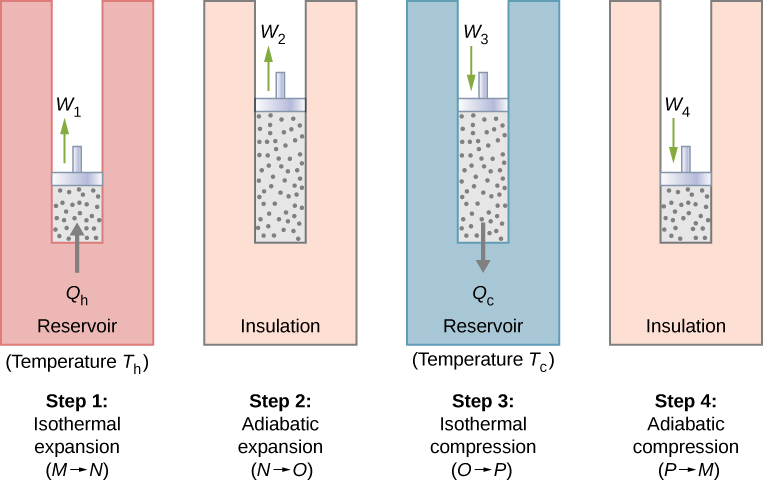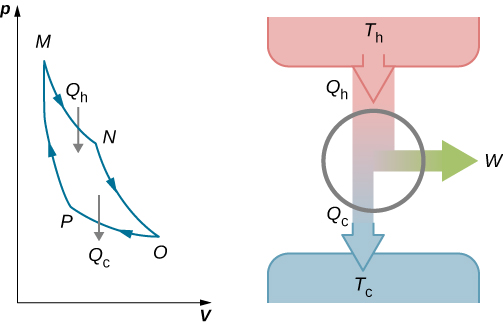# 4.5 The carnot cycle

 Page 1 / 7
• Describe the Carnot cycle with the roles of all four processes involved
• Outline the Carnot principle and its implications
• Demonstrate the equivalence of the Carnot principle and the second law of thermodynamics

In the early 1820s, Sadi Carnot (1786−1832), a French engineer, became interested in improving the efficiencies of practical heat engines. In 1824, his studies led him to propose a hypothetical working cycle with the highest possible efficiency between the same two reservoirs, known now as the Carnot cycle    . An engine operating in this cycle is called a Carnot engine    . The Carnot cycle is of special importance for a variety of reasons. At a practical level, this cycle represents a reversible model for the steam power plant and the refrigerator or heat pump. Yet, it is also very important theoretically, for it plays a major role in the development of another important statement of the second law of thermodynamics. Finally, because only two reservoirs are involved in its operation, it can be used along with the second law of thermodynamics to define an absolute temperature scale that is truly independent of any substance used for temperature measurement.

With an ideal gas as the working substance, the steps of the Carnot cycle, as represented by [link] , are as follows.

1. Isothermal expansion. The gas is placed in thermal contact with a heat reservoir at a temperature ${T}_{\text{h}}.$ The gas absorbs heat ${Q}_{\text{h}}$ from the heat reservoir and is allowed to expand isothermally, doing work ${W}_{1}.$ Because the internal energy ${E}_{\text{int}}$ of an ideal gas is a function of the temperature only, the change of the internal energy is zero, that is, $\text{Δ}{E}_{\text{int}}=0$ during this isothermal expansion. With the first law of thermodynamics, $\text{Δ}{E}_{\text{int}}=Q-W,$ we find that the heat absorbed by the gas is
${Q}_{\text{h}}={W}_{1}=nR{T}_{\text{h}}\phantom{\rule{0.2em}{0ex}}\text{ln}\frac{{V}_{N}}{{V}_{M}}.$The four processes of the Carnot cycle. The working substance is assumed to be an ideal gas whose thermodynamic path MNOP is represented in [link] .The total work done by the gas in the Carnot cycle is shown and given by the area enclosed by the loop MNOPM .
2. Adiabatic expansion . The gas is thermally isolated and allowed to expand further, doing work ${W}_{2}.$ Because this expansion is adiabatic, the temperature of the gas falls—in this case, from ${T}_{\text{h}\phantom{\rule{0.2em}{0ex}}}\text{to}\phantom{\rule{0.2em}{0ex}}{T}_{\text{c}}.$ From $p{V}^{\gamma }=\phantom{\rule{0.2em}{0ex}}\text{constant}$ and the equation of state for an ideal gas, $pV=nRT$ , we have
$T{V}^{\text{γ}\phantom{\rule{0.2em}{0ex}}\text{−}\phantom{\rule{0.2em}{0ex}}\text{1}}=\text{constant},$

so that
${T}_{\text{h}}{V}_{N}{}^{\gamma -1}={T}_{\text{c}}{V}_{O}{}^{\gamma -1}.$
3. Isothermal compression . The gas is placed in thermal contact with a cold reservoir at temperature ${T}_{\text{c}}$ and compressed isothermally. During this process, work ${W}_{3}$ is done on the gas and it gives up heat ${Q}_{\text{c}}$ to the cold reservoir. The reasoning used in step 1 now yields
${Q}_{\text{c}}=nR{T}_{\text{c}}\phantom{\rule{0.2em}{0ex}}\text{ln}\frac{{V}_{O}}{{V}_{P}},$

where ${Q}_{\text{c}}$ is the heat dumped to the cold reservoir by the gas.
4. Adiabatic compression . The gas is thermally isolated and returned to its initial state by compression. In this process, work ${W}_{4}$ is done on the gas. Because the compression is adiabatic, the temperature of the gas rises—from ${T}_{\text{c}}\phantom{\rule{0.2em}{0ex}}\text{to}\phantom{\rule{0.2em}{0ex}}{T}_{\text{h}}$ in this particular case. The reasoning of step 2 now gives
${T}_{\text{c}}{V}_{P}{}^{\gamma -1}={T}_{\text{h}}{V}_{M}{}^{\gamma -1}.$

The total work done by the gas in the Carnot cycle is given by
$W={W}_{1}+{W}_{2}-{W}_{3}-{W}_{4}.$

what is unit
is electric field directly proportional to the squared of a distance
The bullet 2.00cm long is fired at 420/s and passes straight through a 10.0 cm thick board existing at 280 m/s.What is the average acceleration of the bullet through the board?
FAUSTINA
an unstretched spring is 12cm long .A load of 5N stretched it to 15cm .how long will it be under a load of 15N?
Morapeli
hi
Africa
hi
Benjamin
Benjamin how are u are u a freshman in the university
Africa
like 100 level
Africa
yes sir
Benjamin
l need a physics tutor
Benjamin
I think the best tutor is God and organic tutor in YouTube that guy is good
Africa
me too 100level
Africa
wow nice
Benjamin
from Nigeria and u
Africa
I am from Nigeria and u wow nice that something I use to always say
Africa
am from Ghana
Benjamin
ok
Africa
studying what
Africa
Compare the electric flux through the surface of a cube of side length a that has a charge q at its center to the flux through a spherical surface of radius a with a charge q at its center.
please I want to know how to solve increase in length
Ujah
Why a charged capacitor has potential difference but not emf
what is the dimension symbol of temperature?
what is the dimension symbol of temperature?
Keren
what's the meaning of enthalpy in terms of latent heat, internal energy, phase change
how to convert Kelvin to centigrade
what is the s, p, d, f in this table
Sangeetha
s, p, d, f in this table
Sangeetha
what kind of table this
Vengata
what are waves
In physics, mathematics, and related fields, a wave is a propagating dynamic disturbance (change from equilibrium) of one or more quantities
Discuss how would orient a planar surface of area A in a uniform electric field of magnitude E0 to obtain (a) the maximum flux and (b) the minimum flux through the area.
I'm just doing the first 3 with this message. but thankyou for the time your obviously intending to support us with. viva la accumulation
Marcel
Find the net capacitance of the combination of series and parallel capacitors shown belo
what is ohm?
calculate ideal gas pressure of 0.300mol,v=2L T=40°c
what is principle of superposition
what are questions that are likely to come out during exam

#### Get Jobilize Job Search Mobile App in your pocket Now!By OpenStaxBy OpenStaxBy OpenStaxBy Robert MorrisBy Olivia D'AmbrogioBy OpenStaxBy OpenStaxBy OpenStaxBy Courntey HubBy OpenStax